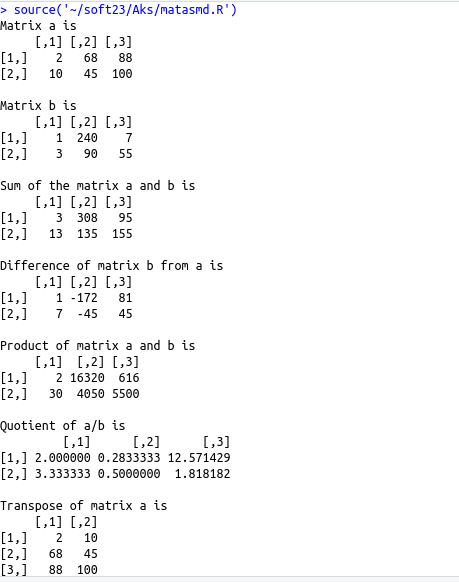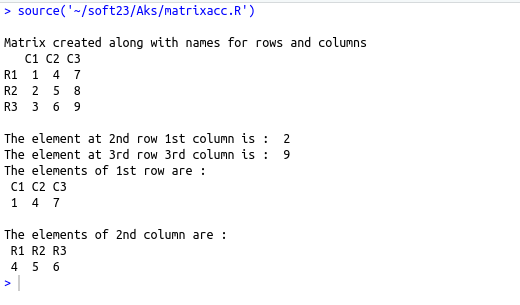• #5, First Floor, 4th Street Dr. Subbarayan Nagar Kodambakkam, Chennai-600 024 Landmark : Samiyar Madam
• pro@slogix.in
• +91- 81240 01111

Explain Matrices concept in R

Description

To understand the matrix concept and its computation.

Process
• Matrix is a rectangular array of elements arranged in rows and columns
• The elements of a matrix belongs to the same atomic type
• The elements of the matrix can be created as character or Logical too but only numeric type matrices are used for mathematical computations

Creating a matrix :
Matrix is created using the function matrix() by passing vector as an argument to it
Syntax :
M=matrix(c(),nrow,ncol,byrow=TRUE,dimnames)

• c() – Vector function to pass the elements
• nrow – No of rows in the matrix
• ncol – No of columns in the matrix
• byrow – If true the elements are filled row by row.If false elements are filled column by column.By default it is FALSE
• dimnames – this parameter is used to give names to rows and columns.The names are given as list

Accessing matrix elements :

• The elements of the matrix can be accessed by their row number and column number as indexing value.

Example : d[1,2],d[1,]d[,2]

D[1,2] – element in 16first row second column

D[1,] – Elements of first row

D[,2] – Elements of second column

Matrix computations :

Mathematical operations can be done in matrices whose result will also be a matrix.

Note that the matrices taken for computations must be of same number of rows and columns.

Transpose of a matrix :

The transpose of a given matrix can be found by using the t() by passing the matrix to it.

M=t(n) where n is a matrix

Sapmle Code

Source code :(Matrix creation)
#matrix
a=matrix(c(1:6),nrow=162,ncol=3,byrow=TRUE)
cat(“Matrix created by filling element row by row\n”)
print(a)
b=matrix(c(1:6),nrow=2,ncol=3)
cat(“\nMatrix created by filling element column by column\n”)
print(b)
rownames=c(“R1″,”R2″,”R3”)
colnames=c(“C1″,”C2″,”C3”)
d=matrix(c(1:9),nrow=3,ncol=3,dimnames=list(rownames,colnames))
cat(“\nMatrix created along with names for rows and columns\n”)
print(d)

Source code :(Accessing elements)
#accessing elements of matrix
rownames=c(“R1″,”R2″,”R3”)
colnames=c(“C1″,”C2″,”C3”)
d=matrix(c(1:9),nrow=3,ncol=3,dimnames=list(rownames,colnames))
cat(“\nMatrix created along with names for rows and columns\n”)
print(d)
cat(“\nThe element at 2nd row 1st column is : “,d[2,1])
cat(“\nThe element at 3rd row 3rd column is : “,d[3,3])
cat(“\nThe elements of 1st row are :\n “)
print(d[1,])
cat(“\nThe elements of 2nd column are :\n “)
print(d[,2])
Source code :(Matrix computations)
a=matrix(c(2,10,68,45,88,100),nrow=2)
b=matrix(c(1,3,240,90,7,55),nrow=2)
cat(“Matrix a is\n”)
print(a)
cat(“\nMatrix b is\n”)
print(b)
cat(“\nSum of the matrix a and b is\n”)
print(a+b)
cat(“\nDifference of matrix b from a is\n”)
print(a-b)
cat(“\nProduct of matrix a and b is \n”)
print(a*b)
cat(“\nQuotient of a/b is\n”)
print(a/b)
cat(“\nTranspose of matrix a is\n”)
print(t(a))

Screenshots